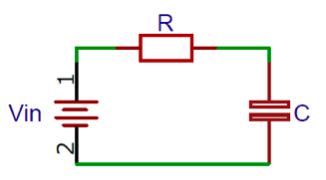# Time Constant Calculator

 Capacitance FmFµFnFpFfF Load Resistance ΩkΩMΩGΩ Voltage VkVmVµV

### OUTPUT

 Seconds s Joules J

This calculator is designed to calculate the capacitor charge time and energy, using the supply voltage, load resistance and Capacitance of the capacitor. The Time Constant Calculator is also sometimes referred to as RC filter calculator or Capacitor charge time calculator, since it is very useful when calculating capacitor value for RC filter or when calculating the energy stored in a capacitor.

The RC time constant is a measure that helps to figure out how much time it will take a capacitor to charge to a certain level. The time taken by a capacitor to reach a certain level is defined by the size of the capacitor and the resistance of the circuit. The RC time constant, also called tau, is equal to the product of the circuit resistance and the circuit capacitance. The RC circuit is a basic circuit in which a resistor of resistance R and a capacitor of capacitance C are connected in a series as shown below.How to Calculate Time Constant & Energy Stored:

Time constant (τ) can be determined from the values of capacitance (C) and load resistance (R). Energy stored on a capacitor (E) can be determined voltage (V) and capacitance:

```τ = R×C

E = CV2/2```

Where:

Voltage (V) = Input voltage to the capacitor in volts

Capacitance (C) = Capacitance in micro-farads

Load Resistance (RL) = Resistance in ohms

Time Constant (τ) = Time Constant in seconds

Energy (E) = Energy stored in capacitor in joules